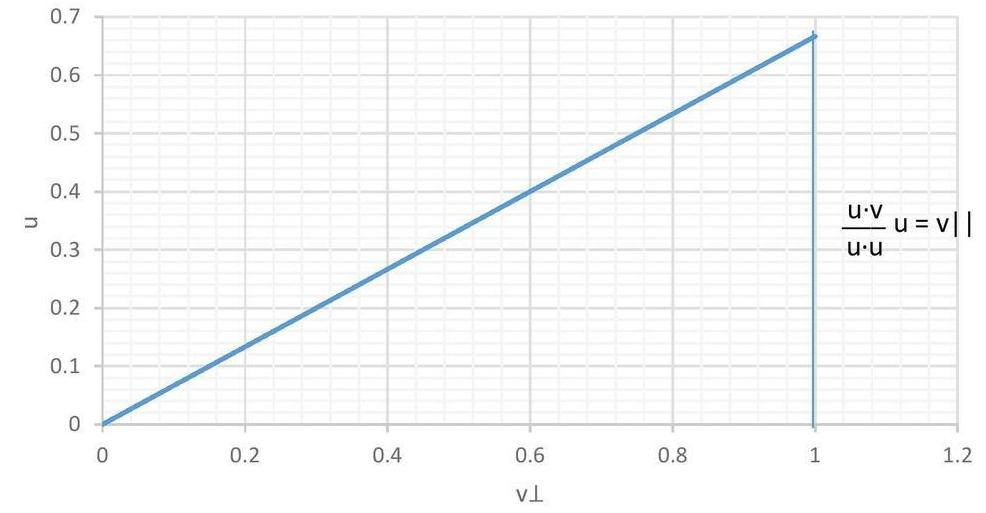$$\newcommand{\id}{\mathrm{id}}$$ $$\newcommand{\Span}{\mathrm{span}}$$ $$\newcommand{\kernel}{\mathrm{null}\,}$$ $$\newcommand{\range}{\mathrm{range}\,}$$ $$\newcommand{\RealPart}{\mathrm{Re}}$$ $$\newcommand{\ImaginaryPart}{\mathrm{Im}}$$ $$\newcommand{\Argument}{\mathrm{Arg}}$$ $$\newcommand{\norm}{\| #1 \|}$$ $$\newcommand{\inner}{\langle #1, #2 \rangle}$$ $$\newcommand{\Span}{\mathrm{span}}$$

# 14.4: Gram-Schmidt and Orthogonal Complements

$$\newcommand{\vecs}{\overset { \rightharpoonup} {\mathbf{#1}} }$$ $$\newcommand{\vecd}{\overset{-\!-\!\rightharpoonup}{\vphantom{a}\smash {#1}}}$$$$\newcommand{\id}{\mathrm{id}}$$ $$\newcommand{\Span}{\mathrm{span}}$$ $$\newcommand{\kernel}{\mathrm{null}\,}$$ $$\newcommand{\range}{\mathrm{range}\,}$$ $$\newcommand{\RealPart}{\mathrm{Re}}$$ $$\newcommand{\ImaginaryPart}{\mathrm{Im}}$$ $$\newcommand{\Argument}{\mathrm{Arg}}$$ $$\newcommand{\norm}{\| #1 \|}$$ $$\newcommand{\inner}{\langle #1, #2 \rangle}$$ $$\newcommand{\Span}{\mathrm{span}}$$ $$\newcommand{\id}{\mathrm{id}}$$ $$\newcommand{\Span}{\mathrm{span}}$$ $$\newcommand{\kernel}{\mathrm{null}\,}$$ $$\newcommand{\range}{\mathrm{range}\,}$$ $$\newcommand{\RealPart}{\mathrm{Re}}$$ $$\newcommand{\ImaginaryPart}{\mathrm{Im}}$$ $$\newcommand{\Argument}{\mathrm{Arg}}$$ $$\newcommand{\norm}{\| #1 \|}$$ $$\newcommand{\inner}{\langle #1, #2 \rangle}$$ $$\newcommand{\Span}{\mathrm{span}}$$

Given a vector $$v$$ and some other vector $$u$$ not in $${\rm span}\, \{v\}$$, we can construct a new vector: $$v^{\perp}:=v-\dfrac{u\cdot v}{u\cdot u}\, u\, .$$This new vector $$v^{\perp}$$ is orthogonal to $$u$$ because

$u\cdot v^{\perp} = u\cdot v - \dfrac{u\cdot v}{u\cdot u}u\cdot u = 0.$

Hence, $$\{u, v^{\perp}\}$$ is an orthogonal basis for $$span \{u,v\}$$. When $$v$$ is not parallel to $$u$$, $$v^{\perp} \neq 0$$, and normalizing these vectors we obtain $$\{\dfrac{u}{|u|}, \dfrac{v^{\perp}}{|v^{\perp}|}\}$$, an orthonormal basis for the vector space $${\rm span}\, \{u,v\}$$.

Sometimes we write $$v = v^{\perp} + v^{\parallel}$$ where:

\begin{eqnarray*}
v^{\perp} &=& v-\dfrac{u\cdot v}{u\cdot u}u \\
v^{\parallel} &=& \phantom{v-}\dfrac{u\cdot v}{u\cdot u}u.
\end{eqnarray*}

This is called an $$\textit{orthogonal decomposition}$$ because we have decomposed $$v$$ into a sum of orthogonal vectors. This decomposition depends on $$u$$; if we change the direction of $$u$$ we change $$v^{\perp}$$ and $$v^{\parallel}$$.

If $$u$$, $$v$$ are linearly independent vectors in $$\Re^{3}$$, then the set $$\{u, v^{\perp}, u\times v^{\perp} \}$$ would be an orthogonal basis for $$\Re^{3}$$. This set could then be normalized by dividing each vector by its length to obtain an orthonormal basis.

However, it often occurs that we are interested in vector spaces with dimension greater than $$3$$, and must resort to craftier means than cross products to obtain an orthogonal basis.

Given a third vector $$w$$, we should first check that $$w$$ does not lie in the span of $$u$$ and $$v$$, $$\textit{i.e.}$$, check that $$u,v$$ and $$w$$ are linearly independent. If it does not, we then can define:

$w^{\perp} = w - \dfrac{u\cdot w}{u\cdot u}\,u - \dfrac{v^{\perp}\cdot w}{v^{\perp}\cdot v^{\perp}}\,v^{\perp}.$

We can check that $$u \cdot w^{\perp}$$ and $$v^{\perp} \cdot w^{\perp}$$ are both zero:

\begin{align*}
u \cdot w^{\perp}&=u \cdot \left(w - \dfrac{u\cdot w}{u\cdot u}\,u - \dfrac{v^{\perp}\cdot w}{v^{\perp}\cdot v^{\perp}}\,v^{\perp} \right)\\&= u\cdot w - \dfrac{u \cdot w}{u \cdot u}u \cdot u - \dfrac{v^{\perp} \cdot w}{v^{\perp} \cdot v^{\perp}} u \cdot v^{\perp} \\
&=u\cdot w-u\cdot w-\dfrac{v^{\perp} \cdot w}{v^{\perp} \cdot v^{\perp}} u \cdot v^{\perp}\ =\ 0
\end{align*}

since $$u$$ is orthogonal to $$v^{\perp}$$, and

\begin{align*}
v^{\perp} \cdot w^{\perp}&=v^{\perp} \cdot \left(w - \dfrac{u\cdot w}{u\cdot u}\,u - \dfrac{v^{\perp}\cdot w}{v^{\perp}\cdot v^{\perp}}\,v^{\perp} \right)\\ &=v^{\perp}\cdot w - \dfrac{u \cdot w}{u \cdot u}v^{\perp} \cdot u - \dfrac{v^{\perp} \cdot w}{v^{\perp} \cdot v^{\perp}} v^{\perp} \cdot v^{\perp} \\
&=v^{\perp}\cdot w-\dfrac{u \cdot w}{u \cdot u}v^{\perp} \cdot u - v^{\perp} \cdot w\ =\ 0
\end{align*}

because $$u$$ is orthogonal to $$v^{\perp}$$. Since $$w^{\perp}$$ is orthogonal to both $$u$$ and $$v^{\perp}$$, we have that $$\{u,v^{\perp},w^{\perp} \}$$ is an orthogonal basis for $$span \{u,v,w\}$$.

## 14.4.1: The Gram-Schmidt Procedure

In fact, given a set $$\{v_{1}, v_{2}, \ldots \}$$ of linearly independent vectors, we can define an orthogonal basis for $$span \{v_{1},v_{2}, \ldots \}$$ consisting of the following vectors:

\begin{eqnarray*}
v_{1}^{\perp}&:=&v_{1} \\
v_{2}^{\perp} &:=& v_{2} - \dfrac{v_{1}^{\perp}\cdot v_{2}}{v_{1}^{\perp}\cdot v_{1}^{\perp}}\,v_{1}^{\perp} \\
v_{3}^{\perp} &:=& v_{3} - \dfrac{v_{1}^{\perp}\cdot v_{3}}{v_{1}^{\perp}\cdot v_{1}^{\perp}}\,v_{1}^{\perp} - \dfrac{v_{2}^{\perp}\cdot v_{3}}{v_{2}^{\perp}\cdot v_{2}^{\perp}}\,v_{2}^{\perp}\\
&\vdots& \\
v_{i}^{\perp} &=& v_{i} - \sum_{j<i} \dfrac{v_{j}^{\perp}\cdot v_{i}}{v_{j}^{\perp}\cdot v_{j}^{\perp}}\,v_{j}^{\perp} \\
&:=& v_{i} - \dfrac{v_{1}^{\perp}\cdot v_{i}}{v_{1}^{\perp}\cdot v_{1}^{\perp}}\,v_{1}^{\perp}
- \dfrac{v_{2}^{\perp}\cdot v_{i}}{v_{2}^{\perp}\cdot v_{2}^{\perp}}\,v_{2}^{\perp} -\cdots
- \dfrac{v_{i-1}^{\perp}\cdot v_{i}}{v_{i-1}^{\perp}\cdot v_{i-1}^{\perp}}\,v_{i-1}^{\perp}\\
&\vdots& \\
\end{eqnarray*}

Notice that each $$v_{i}^{\perp}$$ here depends on $$v_{j}^{\perp}$$ for every $$j<i$$. This allows us to inductively/algorithmically build up a linearly independent, orthogonal set of vectors $$\{v_{1}^{\perp},v_{2}^{\perp}, \ldots \}$$ such that $$span \{v_{1}^{\perp},v_{2}^{\perp}, \ldots \}=span \{v_{1}, v_{2}, \ldots \}$$. That is, an orthogonal basis for the latter vector space. This algorithm is called the $$\textit{Gram--Schmidt orthogonalization procedure}$$--Gram worked at a Danish insurance company over one hundred years ago, Schmidt was a student of Hilbert (the famous German mathmatician).

Example 124

We'll obtain an orthogonal basis for $$\Re^{3}$$ by appling Gram-Schmidt to the linearly independent set $$\{ v_{1}=\begin{pmatrix}1\\1\\0\end{pmatrix}, v_{2}=\begin{pmatrix}1\\1\\1\end{pmatrix},v_{3}=\begin{pmatrix}3\\1\\1\end{pmatrix}\}$$. First, we set $$v_{1}^{\perp}:=v_{1}$$. Then:

\begin{eqnarray*}
v_{2}^{\perp}&=& \begin{pmatrix}1\\1\\1\end{pmatrix} - \dfrac{2}{2}\begin{pmatrix}1\\1\\0\end{pmatrix} = \begin{pmatrix}0\\0\\1\end{pmatrix} \\
v_{3}^{\perp}&=& \begin{pmatrix}3\\1\\1\end{pmatrix} - \dfrac{4}{2}\begin{pmatrix}1\\1\\0\end{pmatrix} - \dfrac{1}{1}\begin{pmatrix}0\\0\\1\end{pmatrix} = \begin{pmatrix}1\\-1\\0\end{pmatrix}.
\end{eqnarray*}

Then the set

$\left\{ \begin{pmatrix}1\\1\\0\end{pmatrix},\begin{pmatrix}0\\0\\1\end{pmatrix},\begin{pmatrix}1\\-1\\0\end{pmatrix}\right\}$

is an orthogonal basis for $$\Re^{3}$$. To obtain an orthonormal basis, as always we simply divide each of these vectors by its length, yielding:

$\left\{ \begin{pmatrix}\dfrac{1}{\sqrt2}\\\dfrac{1}{\sqrt2}\\0\end{pmatrix},\begin{pmatrix}0\\0\\1\end{pmatrix},\begin{pmatrix}\dfrac{1}{\sqrt2}\\\dfrac{-1}{\sqrt2}\\0\end{pmatrix}\right\}.$